### Vol 17, No 8 (2017) / Yang

Relativistic time transfer for a Mars lander: from Areocentric Coordinate Time to Barycentric Coordinate Time

# Relativistic time transfer for a Mars lander: from Areocentric Coordinate Time to Barycentric Coordinate Time

Yang Wen-Zheng1, 2, Xu De-Wang1, 2, Yu Qing-Shan1, 2, Liu Jie1, 2, Xie Yi1, 2,

School of Astronomy and Space Science, Nanjing University, Nanjing 210093, China
Key Laboratory of Modern Astronomy and Astrophysics, Nanjing University, Ministry of Education, Nanjing 210093, China

† Corresponding author. E-mail: yixie@nju.edu.cn

Abstract: Abstract

As the second step of relativistic time transfer for a Mars lander, we investigate the transformation between Areocentric Coordinate Time (TCA) and Barycentric Coordinate Time (TCB) in the framework of IAU Resolutions. TCA is a local time scale for Mars, which is analogous to the Geocentric Coordinate Time (TCG) for Earth. This transformation has two parts: contributions associated with gravitational bodies and those depending on the position of the lander. After setting the instability of an onboard clock to 10−13 and considering that the uncertainty in time is about 3.2 microseconds after one Earth year, we find that the contributions of the Sun, Mars, Jupiter and Saturn in the leading term associated with these bodies can reach a level exceeding the threshold and must be taken into account. Other terms can be safely ignored in this transformation for a Mars lander.

Keywords: reference systems;time;space vehicles

## 1 Introduction

Time transfer plays an important role in every deep space mission. Einstein’s general relativity (GR) (Iorio ; Debono & Smoot ) has been an inevitable part of time transfer in both a theoretical sense (Misner et al. ; Landau & Lifshitz ) and in practice (Petit & Wolf ; Nelson , ). In the framework of GR, there are two kinds of time: proper time and coordinate time, which are connected by a 4-dimensional spacetime interval, so that clock synchronization and time/frequency transfer for deep space tracking and navigation have been considerably changed (Moyer ; Thornton & Border ). In the future, time transfer might be used to test GR and alternative theories of gravity (e.g., Wolf et al. ; Christophe et al. ; Schiller et al. ; Deng & Xie ; Christophe et al. ; Angélil et al. ; Delva et al. ).

In this work, as a continuation of the time transfer for a Mars lander (Xu et al. ), we will focus on the relativistic transformation from Areocentric Coordinate Time (TCA) to Barycentric Coordinate Time (TCB), where TCA is the time coordinate of the Areocentric Celestial Reference System (ACRS). ACRS is introduced to describe events that happen in the vicinity of Mars and its definition is consistent with the International Astronomical Union (IAU) 2000 Resolutions (Soffel et al. ) and 2006 Resolution B2 1 (see Xu et al. , for more details). This transformation is the second step in the whole procedure of time transfer. Although this work is devoted to time transfer for a Mars lander, the major part of the transformation from TCA to TCB only depends on the position and velocity of gravitational bodies in the Solar System and it can also be applied to a Mars orbiter (Deng ; Pan & Xie , , ). In the practical realization of the time transfer link, we will also consider GR effects on the propagation of electromagnetic waves from Mars to Earth and vice-versa in the post-Newtonian field of the Solar System and the effects of the post-Newtonian field of Mars itself on a Mars probe (Iorio , , ; Oberst et al. ; Turyshev et al. ), which will be one of our next moves.

Following engineering settings of the previous work (Xu et al. ), we also consider a clock onboard the Mars lander with instability at the level of 10−13 so that the uncertainty in time is about 3.2 microsecond (μs) after linear drifting for one (Earth) year. Therefore, any effects below these two thresholds will be dropped.

The paper is organized as follows. In Section 2, we describe the relativistic transformation between TCA and TCB based on IAU 2000 Resolutions (Soffel et al. ). This transformation has two parts: contributions associated with gravitational bodies in the Solar System and terms directly depending on the position of the lander. Sections 3 and 4 are respectively devoted to examining these two contributions. We summarize the results in Section 5.

## 2 Transformation from TCA to TCB

According to the IAU Resolutions (Soffel et al. ), the post-Newtonian transformation between TCA and TCB can be written in a linear form as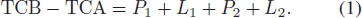Here, P 1 and P 2 are contributions associated with gravitational bodies in the Solar System and they are independent of the position and velocity of the Mars lander. P 1,2 are in the forms of integrals as (Soffel et al. )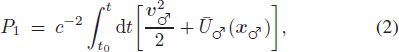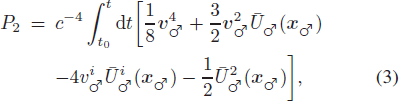where c is the speed of light; t is the coordinate time in the scale of TCB;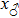and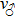are respectively the position and velocity of Mars in the Barycentric Celestial Reference System (BCRS). In Equations (2) and (3),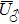and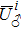are respectively the Newtonian gravitational potential and a vector potential, both of which are generated by gravitational bodies except Mars; their expressions read as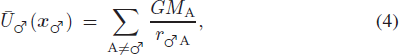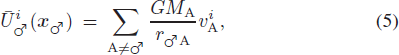where M A is the mass of body A;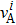is the velocity of body A in the BCRS; and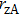is the distance between Mars and body A. Since the distance between bodies is much larger than their characteristic lengths in the Solar System, the non-spherical parts of their gravitational fields can be neglected in this case. It is worth mentioning that all of the variables in the integrands of P 1 and P 2 are functions of TCB, although such a dependence is not shown explicitly.

Unlike P 1 and P 2, the other parts of TCB-TCA, L 1 and L 2, directly depend on the position of the Mars lander as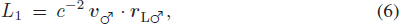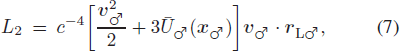where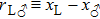and x L is the position of the lander in the BCRS.

3 Contributions of P 1 and P 2

In order to calculate the contributions of P 1 and P 2, we have to know the positions and velocities of bodies in the Solar System. In this investigation, we consider the Sun, eight planets and the three largest asteroids (Ceres, Pallas and Vesta). We read their positions and velocities with the DE431 ephemeris (Folkner et al. ) from the starting time 2023-Jan-01 to the end point 2024-Jan-01. However, the time coordinate of DE431 is the Barycentric Dynamical Time (TDB), instead of TCB, but they have a linear relation described as (Petit & Luzum )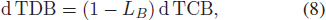where LB = 1.550519768 × 10−8 is a defining constant. Hence, we can rewrite Equations (2) and (3) as (Soffel et al. )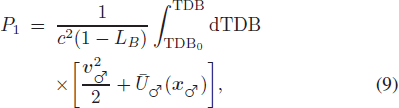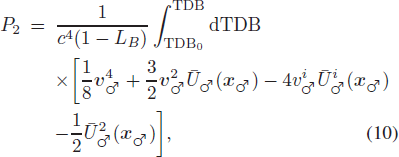where all variables in the above integrands are functions of TDB and can be read directly from DE431.

Figure 1 shows components of P 1 contributed by various bodies and their overall values. We find that P 1 can increase to about 0.3 s after one year and contributions of the Sun, Mars, Jupiter and Saturn can reach the level of more than 3 μs.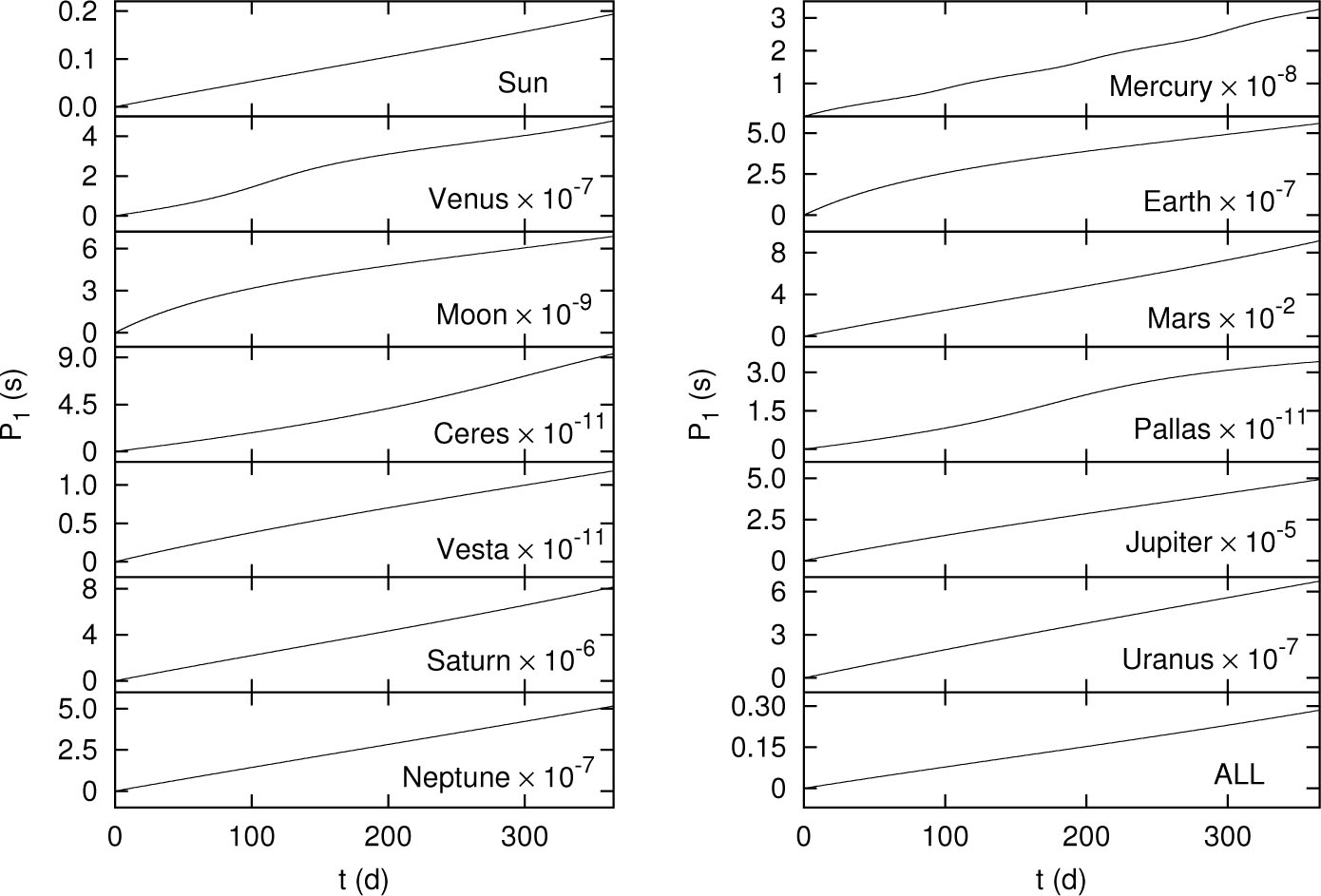Fig. 1 Components of P 1 contributed by various bodies and their overall values (marked by “ALL”).

Figure 2 shows components of P 2 contributed by various bodies and their overall values. Since its overall contribution is about 1 nanosecond (ns), much less than our threshold, we can safely ignore P 2 in this investigation. Like the work of Xu et al. (), we also estimate the contribution of the hypothetical Planet Nine or Telisto (Batygin & Brown ; Brown & Batygin ; Mustill et al. ; Iorio , , ) on P 1 to be about 10 ns, which can be neglected.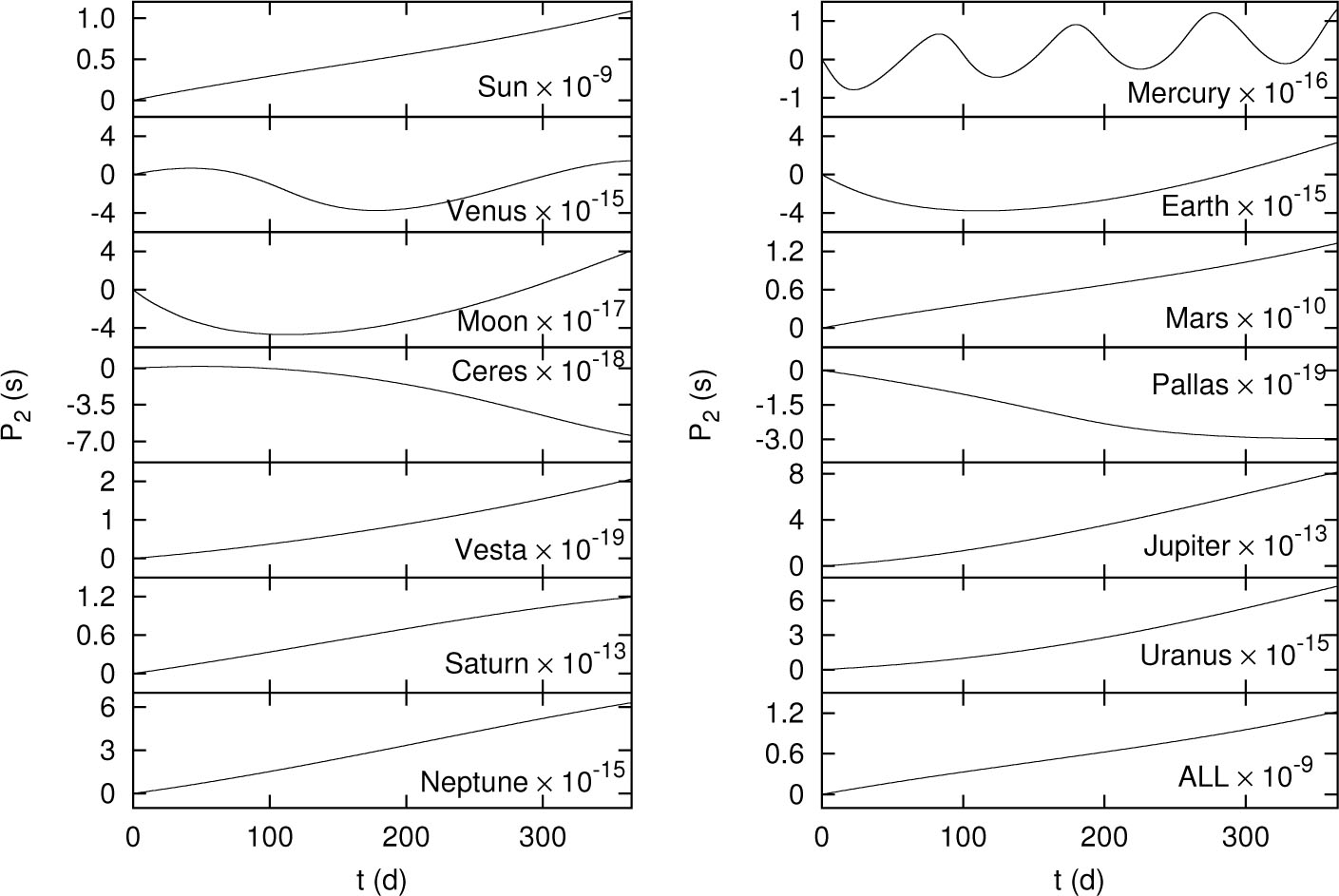Fig. 2 Components of P 2 contributed by various bodies and their overall values (marked by “ALL”).

If the lander has to process the calculation of P 1 for autonomous time transfer, it would be necessary to construct an analytic form to approximate P 1 with sufficient accuracy because the capacity of the onboard computer is expected to be very limited. In principle, we can apply the strategy for constructing time transformations TDB-Terrestrial Time (TT) by using an analytical planetary ephemeris (Fairhead & Bretagnon ) and TCBTCG by Chebyshev polynomial fitting (Fukushima ; Irwin & Fukushima ; Fukushima ) or by nonlinear harmonic decomposition (Harada & Fukushima ). Although the detailed investigation on this will be left as one of our next moves, we will study the properties of P 1 in order to provide some clues for future works. Following the work of Pan & Xie (), we take an nth polynomial to fit P 1 as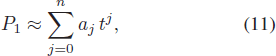and then perform Fourier analysis on its post-fit residuals. In the future, this preliminary procedure will be replaced with a more sophisticated one, such as the approach in the works (Kudryavtsev , ).

Table 1 shows the coefficients aj of the fitted nth polynomial and Figure 3 represents the post-fit residuals. It is found that, for the cases that n = 4 and n = 5, the residuals can decrease to about 30 μs and they can even decrease to several μs during t from 100 d to 300 d.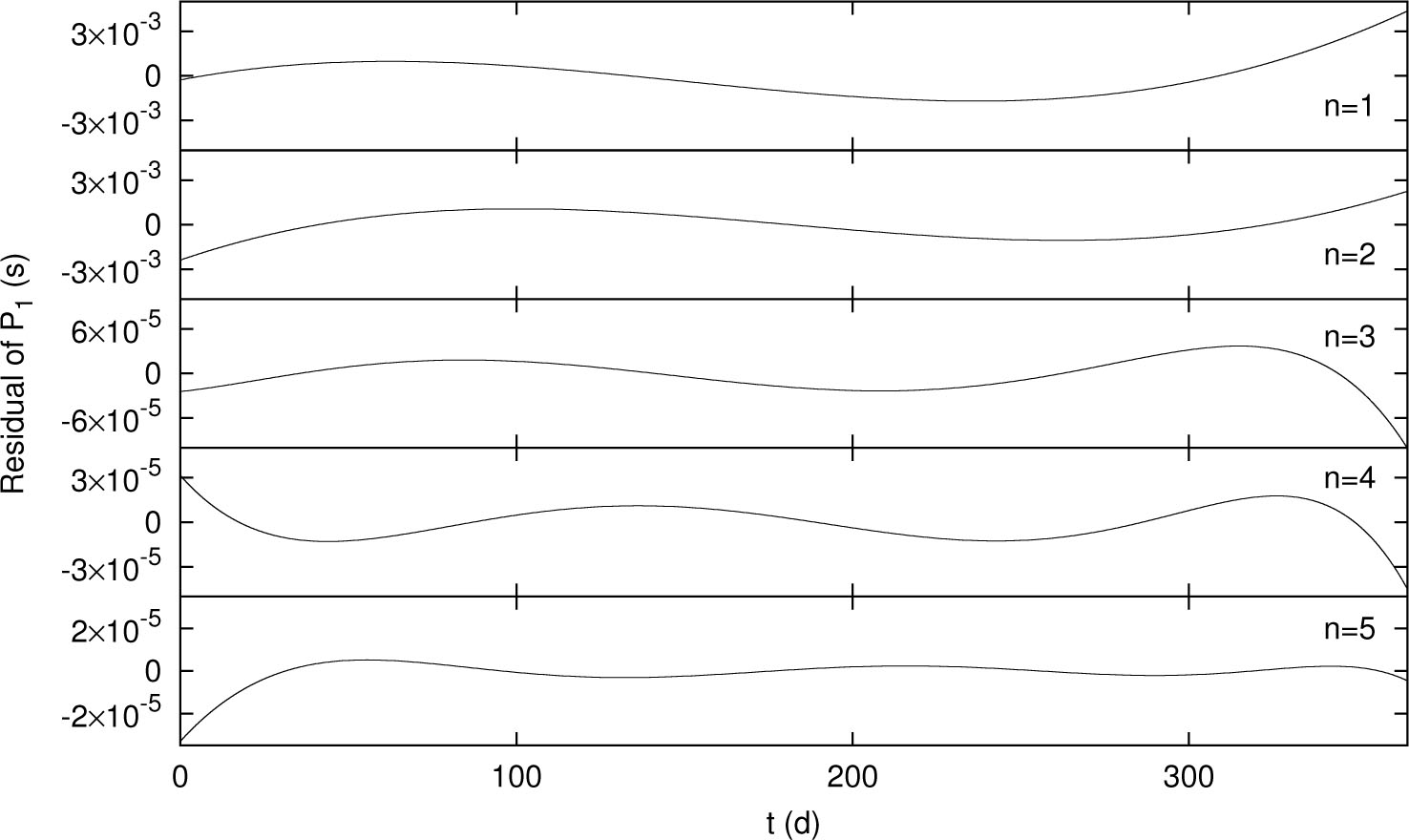Fig. 3 Post-fit residuals of P 1 for the nth polynomial where n = 1, ⋯, 5.
 n aj Value 1 a 0 2.729372422064708 × 10−4 a 1 8.881818856983953 × 10−9 2 a 0 2.387954153226595 × 10−3 a 1 8.479410723073467 × 10−9 a 2 1.276027821887640 × 10−17 3 a 0 2.993148212994718 × 10−5 a 1 9.376722304005195 × 10−9 a 2 −5.837430741133446 × 10−17 a 3 1.503775275456851 × 10−24 4 a 0 −2.586492924316603 × 10−5 a 1 9.412111185814432 × 10−9 a 2 −6.342420286347526 × 10−17 a 3 1.752869730773431 × 10−24 a 4 −3.949366681198948 × 10−33 5 a 0 3.875654970449868 × 10−5 a 1 9.362187216272435 × 10−9 a 2 −5.319681905071191 × 10−17 a 3 9.241183787895206 × 10−25 a 4 2.484444940015867 × 10−32 a 5 −3.587172304388239 × 10−40

Table 1 Coefficients of the fitted nth polynomial

Figure 4 shows frequency spectra of these post-fit residuals. The spectra with n = 1 and n = 3 have distinct peaks, but these peaks are absent in the others. As demonstrated by the work of Pan & Xie (), their spike-like and tip-like shapes do not mean there is any discontinuity at those points, but rather they are caused by the low resolution of the figure.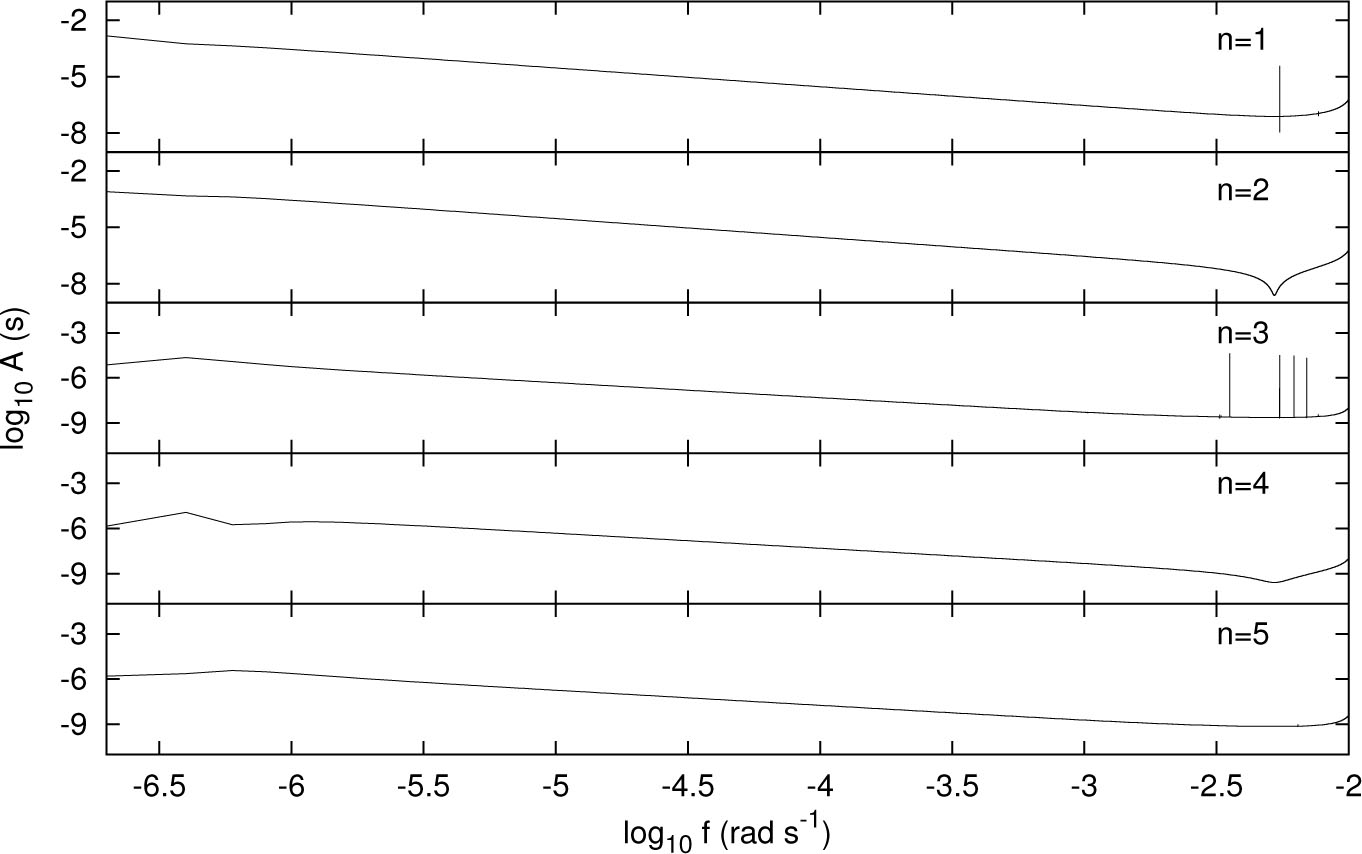Fig. 4 Frequency spectra of the post-fit residuals of P 1 for the nth polynomial where n = 1, ⋯, 5, and where “A” and “f” denote amplitude and frequency, respectively.
4 Contributions of L 1 and L 2

The terms L 1 and L 2 are different from P 1 and P 2 by depending on positions of the lander and their values will not accumulate with time. As a straightforward estimation, we can find that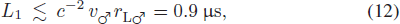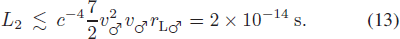Therefore, both of them are below the threshold we set and can be safely ignored in this case. In the above estimation, we take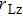as the radius of Mars for a lander. When an orbiter of Mars is considered,can be larger, which makes L 1 close to or bigger than the threshold so that the term L 1 has to be included in the relativistic transformation between TCA and TCB.

## 5 Conclusions

In this paper, we continue the work on time transfer for a Mars lander (Xu et al. ) and investigate its second step that is the relativistic transformation between TCA and TCB. This transformation has two parts: P 1,2 terms associated with the gravitational bodies [see Eqs. (2) and (3)] and L 1,2 terms depending on the position of the lander [see Eqs. (6) and (7)].

After setting the instability of the onboard clock as 10−13, we find that the contributions of the Sun, Mars, Jupiter and Saturn in P 1 can reach the level exceeding the threshold and must be taken into account. Other terms, P 2 and L 1,2, can be safely ignored in this transformation for a Mars lander.

Besides a part of the procedure for time transfer, such a transformation between TCA and TCB might also be used in the model of Doppler tracking of a lander and an orbiter of Mars (Deng & Xie ; Zhang et al. ; Yang et al. ; Xie & Huang ), since the time coordinate of their equations of motion is TCA according to the IAU 2000 Resolutions (Soffel et al. ).When considering much more precise and accurate measurements, the high-order contributions might be included (Deng & Xie ; Deng ; Xie & Huang ; Deng ).

 Angélil R. Saha P. Bondarescu R. et al. 2014 Phys. Rev. D 89 064067 Batygin K. Brown M. E. 2016 AJ 151 22 Brown M. E. Batygin K. 2016 ApJ 824 L23 Christophe B. Andersen P. H. Anderson J. D. et al. 2009 Experimental Astronomy 23 529 Christophe B. Spilker L. J. Anderson J. D. et al. 2012 Experimental Astronomy 34 203 Debono I. Smoot G. F. 2016 Universe 2 23 Delva P. Hees A. Bertone S. Richard E. Wolf P. 2015 Classical and Quantum Gravity 32 232003 Deng X.-M. 2012 RAA (Research in Astronomy and Astrophysics) 12 703 Deng X.-M. 2015 International Journal of Modern Physics D 24 1550056 Deng X.-M. 2016 International Journal of Modern Physics D 25 1650082 Deng X.-M. Xie Y. 2012 Phys. Rev. D 86 044007 Deng X.-M. Xie Y. 2013 MNRAS 431 3236 Deng X.-M. Xie Y. 2014 RAA (Research in Astronomy and Astrophysics) 14 319 Fairhead L. Bretagnon P. 1990 A&A 229 240 Folkner W. M. Williams J. G. Boggs D. H. Park R. S. Kuchynka P. 2014 Interplanet. Netw. Prog. Rep 196 C1 Fukushima T. 1995 A&A 294 895 Fukushima T. 2010 IAU Symposium 261 Relativity in Fundamental Astronomy: Dynamics, Reference Frames, and Data Analysis Klioner S. A. Seidelmann P. K. Soffel M. H. 89 Harada W. Fukushima T. 2003 AJ 126 2557 Iorio L. 2006 Classical and Quantum Gravity 23 5451 Iorio L. 2009 General Relativity and Gravitation 41 1273 Iorio L. 2010 Open Physics 8 509 Iorio L. 2012 Celestial Mechanics and Dynamical Astronomy 112 117 Iorio L. 2014 MNRAS 444 L78 Iorio L. 2015 Universe 1 38 Iorio L. 2017 Ap&SS 362 11 Irwin A. W. Fukushima T. 1999 A&A 348 642 Kudryavtsev S. M. 2007 A&A 471 1069 Kudryavtsev S. M. 2016 MNRAS 456 4015 Landau L. D. Lifshitz E. M. 1975 The Classical Theory of Fields 4 Oxford Pergamon Press Misner C. W. Thorne K. S. Wheeler J. A. 1973 Gravitation San Francisco W.H. Freeman and Co. Moyer T. D. 2005 Formulation for Observed and Computed Values of Deep Space Network Data Types for Navigation 3 John Wiley & Sons Mustill A. J. Raymond S. N. Davies M. B. 2016 MNRAS 460 L109 Nelson R. A. 2007 Frequency Control Symposium, 2007 Joint with the 21st European Frequency and Time Forum IEEE International, IEEE 1278 Nelson R. A. 2011 Metrologia 48 S171 Oberst J. Lainey V. Le Poncin-Lafitte C. et al. 2012 Experimental Astronomy 34 243 Pan J.-Y. Xie Y. 2013 RAA (Research in Astronomy and Astrophysics) 13 1358 Pan J.-Y. Xie Y. 2014 RAA (Research in Astronomy and Astrophysics) 14 233 Pan J.-Y. Xie Y. 2015 RAA (Research in Astronomy and Astrophysics) 15 281 Petit G. Luzum B. 2010 IERS conventions (2010), Tech. rep., DTIC Document Bundesamt für Kartographie und Geodäsie Petit G. Wolf P. 2005 Metrologia 42 S138 Schiller S. Tino G. M. Gill P. et al. 2009 Experimental Astronomy 23 573 Soffel M. Klioner S. A. Petit G. et al. 2003 AJ 126 2687 Thornton C. L. Border J. S. 2005 Radiometric Tracking Techniques for Deep Space Navigation 63 Turyshev S. G. Farr W. Folkner W. M. et al. 2010 Experimental Astronomy 28 209 Wolf P. Bordé C. J. Clairon A. et al. 2009 Experimental Astronomy 23 651 Xie Y. Huang Y. 2015 RAA (Research in Astronomy and Astrophysics) 15 1751 Xu D.-W. Yu Q.-S. Xie Y. 2016 RAA (Research in Astronomy and Astrophysics) 16 155 Yang Z.-S. Han Y.-C. Liu J.-H. et al. 2014 RAA (Research in Astronomy and Astrophysics) 14 1343 Zhang Y.-F. Zhang X.-Z. Liu J.-H. Huang Y. Xie Y. 2014 RAA (Research in Astronomy and Astrophysics) 14 1201
Cite this article: Yang Wen-Zheng, Xu De-Wang, Yu Qing-Shan, Liu Jie, Xie Yi. Relativistic time transfer for a Mars lander: from Areocentric Coordinate Time to Barycentric Coordinate Time. Cancer Biol Med. 2017; 8:088.

### Refbacks

• There are currently no refbacks.